# Team:NDNF China/Model## IntroductionModelling in synthetic biology is a powerful tool that allows us to get a deeper understanding of our system. It guided and assisted the design of our detection system which helped us save a lot of time by avoiding dead-end designs.

In this project, we have designed a genetic switch that can be induced by caffeine. We expected to describe the activation of the caffeine-inducible switch through mathematical modelling to give a quantitative understanding between signal input and output, and thus provide guidance for realistic scenario applications.

## Caffeine circuit design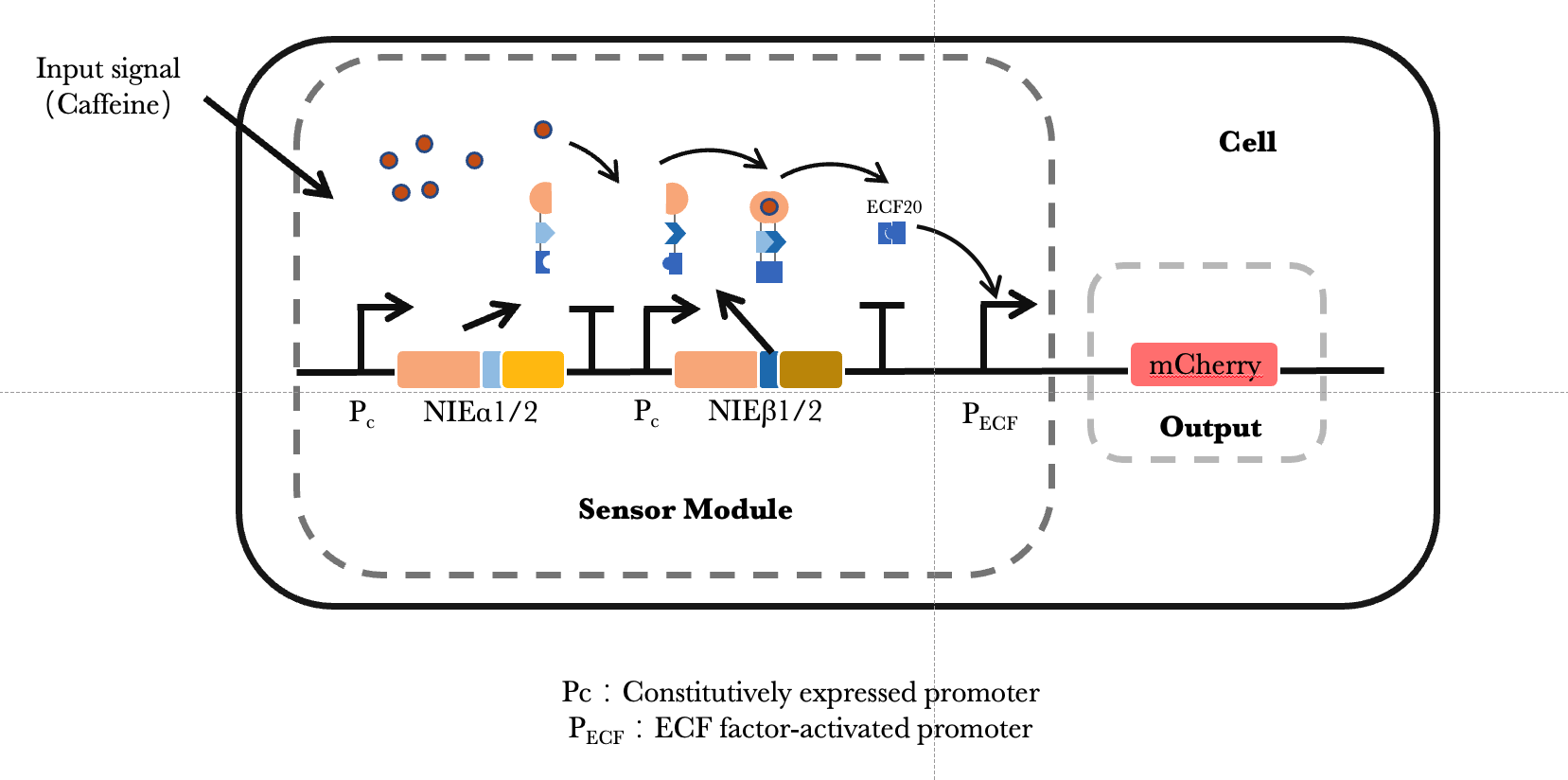1. The caffeine nanobody-intein-ECF20 (NIE) is split separately, and then divided into two parts for expression.

2. The input of caffeine will induce the recombine of split caffeine nanobody-intein-ECF20 (NIE) protein(NIE), and then under the action of the intein, the split ECF20 will be recombined and the completed ECF20 will be released in the cytoplasm. Then the ECF20 will activate the pECF promoter and the expression of corresponding output. See more information, please visit our Proof-Of-Concept Page.

## ODE Equations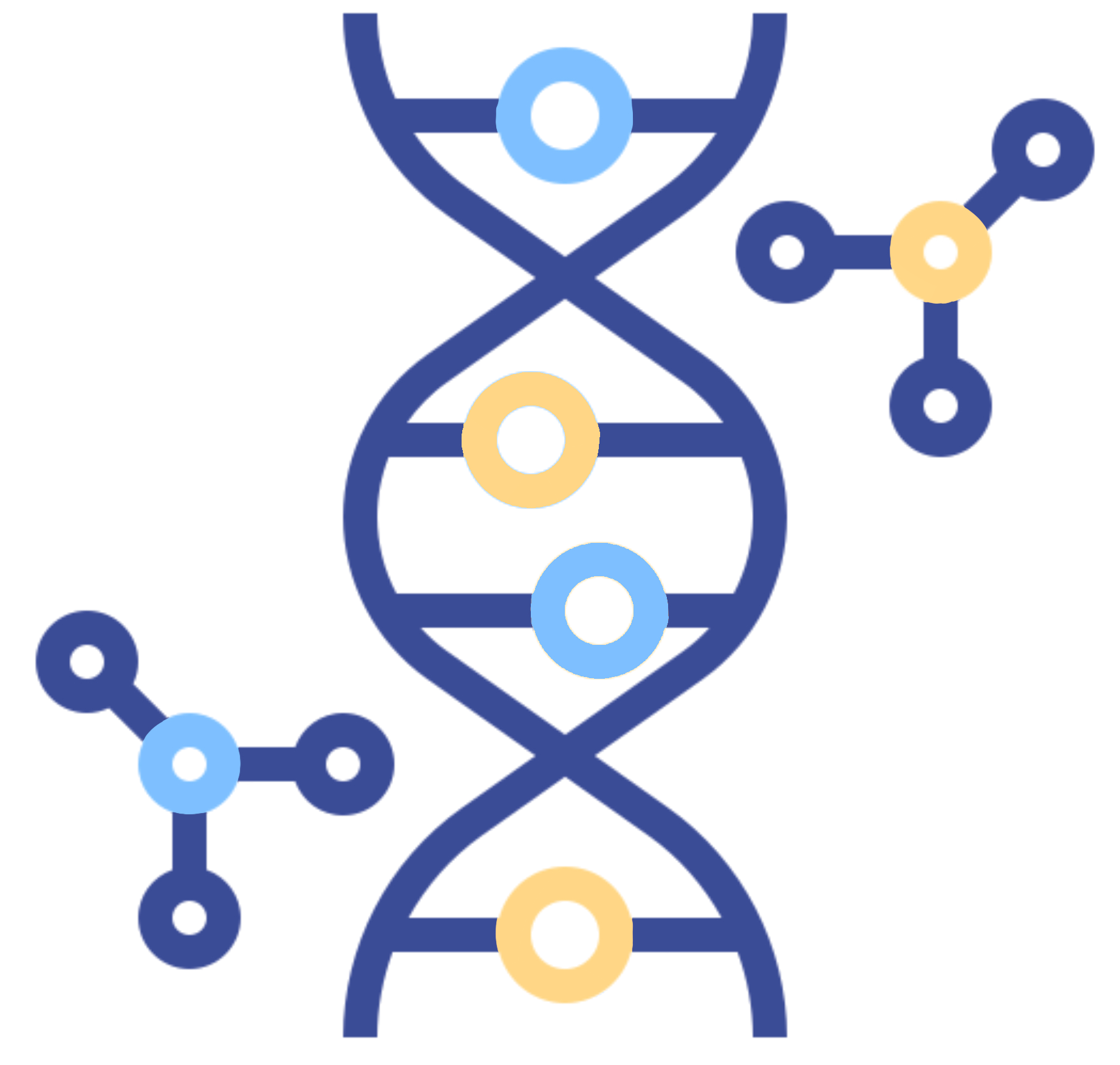### Chemical formulasIt describes the process of combining NIEα(1/2) protein and NIEβ(1/2) protein into NIE complex, and the breakdown of the NIE protein back into two separate parts.It describes the process that NIE binds to caffeine, forming a NIE-caffeine complex, which gradually turns in to ECF20 molecule and NI-Caffeine complex.It describes the process that NIE-caffeine complex gradually turns in to NI complex and Caffeine molecule.

Abbreviations

- NIE: Complex of Nanobody-Intein-ECF20

- NI: Complex of Nanobody-Intein

### Mathematical formula

$$1.\frac{d[P_{NIEα(1/2)}]}{dt}=K_{P_{NIEα(1/2)}}-d_{P_{NIEα(1/2)}}[P_{NIEα(1/2)}]$$

Describe the protein expression of caffeine nanobody - intein -ECF20 (NIE) part α.

$$2.\frac{d[P_{NIEβ(1/2)}]}{dt}=K_{P_{NIEβ(1/2)}}-d_{P_{NIEβ(1/2)}}[P_{NIEβ(1/2)}]$$

Describe the protein expression of caffeine nanobody - intein -ECF20 (NIE) part β.

$$3.\frac{d[P_{NIE}]}{dt}=K_{b1}[P_{NIEα(1/2)}][P_{NIEβ(1/2)}]-K_{b2}[P_{NIE}]-d_{P_{NIE}}[P_{NIE}]-K_{b3}[P_{NIE}][Caffeine]+K_{b4}[P_{NIE-Caffeine}]$$

Describe the protein production changes of caffeine nanobody-intein-ECF20 complex (NIE).

$$4.\frac{d[Caffeine]}{dt}=-K_{b3}[P_{NIE}][Caffeine]+K_{b4}[P_{NIE-Caffeine}]+K_{b6}[P_{NI-Caffeine}]$$

Describe caffeine concentration changes in the environment.

$$5.\frac{d[P_{NI-Caffeine}]}{dt}=K_{b5}[P_{NIE}][Caffeine]-d_{P_{NI-Caffeine}}[P_{NI-Caffeine}]$$

$$6.\frac{d[NIE-Caffeine]}{dt}=K_{b3}[P_{NIE}][Caffeine]-K_{b4}[P_{NIE-Caffeine}]-d_{P_{NIE-Caffeine}}[P_{NIE-Caffeine}]-K_{b5}[P_{NIE-Caffeine}]$$

Describe the protein concentration changes of the NIE-caffeine complex.

$$7. \frac{d[P_{ECF20}]}{dt}=K_{b5}[P_{NIE-Caffeine}]-d_{P_{ECF20}}[P_{ECF20}]$$

Describe changes in the levels of ECF20 protein in the environment.

$$8. \frac{d[M_{mcherry}]}{dt}=K_{M_{mcherry}}\frac{[P_{ECF20}]^n}{[P_{ECF20}]^n+K^n}-d_{M_{mcherry}}[M_{mcherry}]$$

Describe the process of ECF20 activating mCherry mRNA expression.

$$9. \frac{d[P_{mcherry}]}{dt}=K_{P_{mcherry}}[M_{mcherry}]-d_{P_{mcherry}}[P_{mcherry}]$$

Describe the expression of mCherry protein.

### Parameters

$K_{P_{NIEα(1/2)}}$ ：Constant for NIEα(1/2) protein production

$K_{P_{NIEβ(1/2)}}$ ：Constant for NIEβ(1/2) protein production

$d_{P_{NIEα(1/2)}}$ ：Constant for NIEα(1/2) protein degradation

$d_{P_{NIEβ(1/2)}}$：Constant for NIEβ(1/2) protein degradation

$K_{b1}$：Binding constant for the reaction [NIEα1/2] + [NIEβ1/2] —> [NIE]

$K_{b2}$：Binding constant for the reaction [NIE] —> [NIEα1/2] + [NIEβ1/2]

$d_{P_{NIE}}$：Constant for NIE protein degradation

$K_{b5}$：Binding constant for the reaction [NIE] + [Caffeine] —> [ECF20] + [NI-Caffeine]

$K_{b6}$：Binding constant for the reaction [NI-Caffeine] —> [NI] + [Caffeine]

$d_{P_{ECF20}}$：Constant for ECF20 protein degradation

$K_{M_{mcherry}}$：Constant for mCherry mRNA production

$K$：Constant for Hill function

$n$：Hill exponent

$d_{M_{mcherry}}$：Constant for mCherry mRNA degradation

$K_{P_{mcherry}}$：Constant for mCherry protein production

$d_{P_{mcherry}}$：Constant for mCherry protein degradation

## Hill Equation Fitting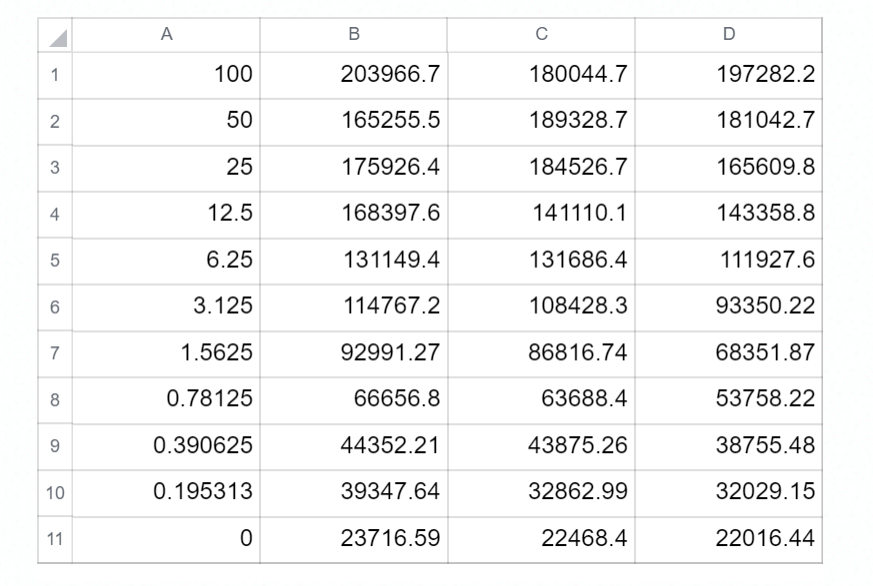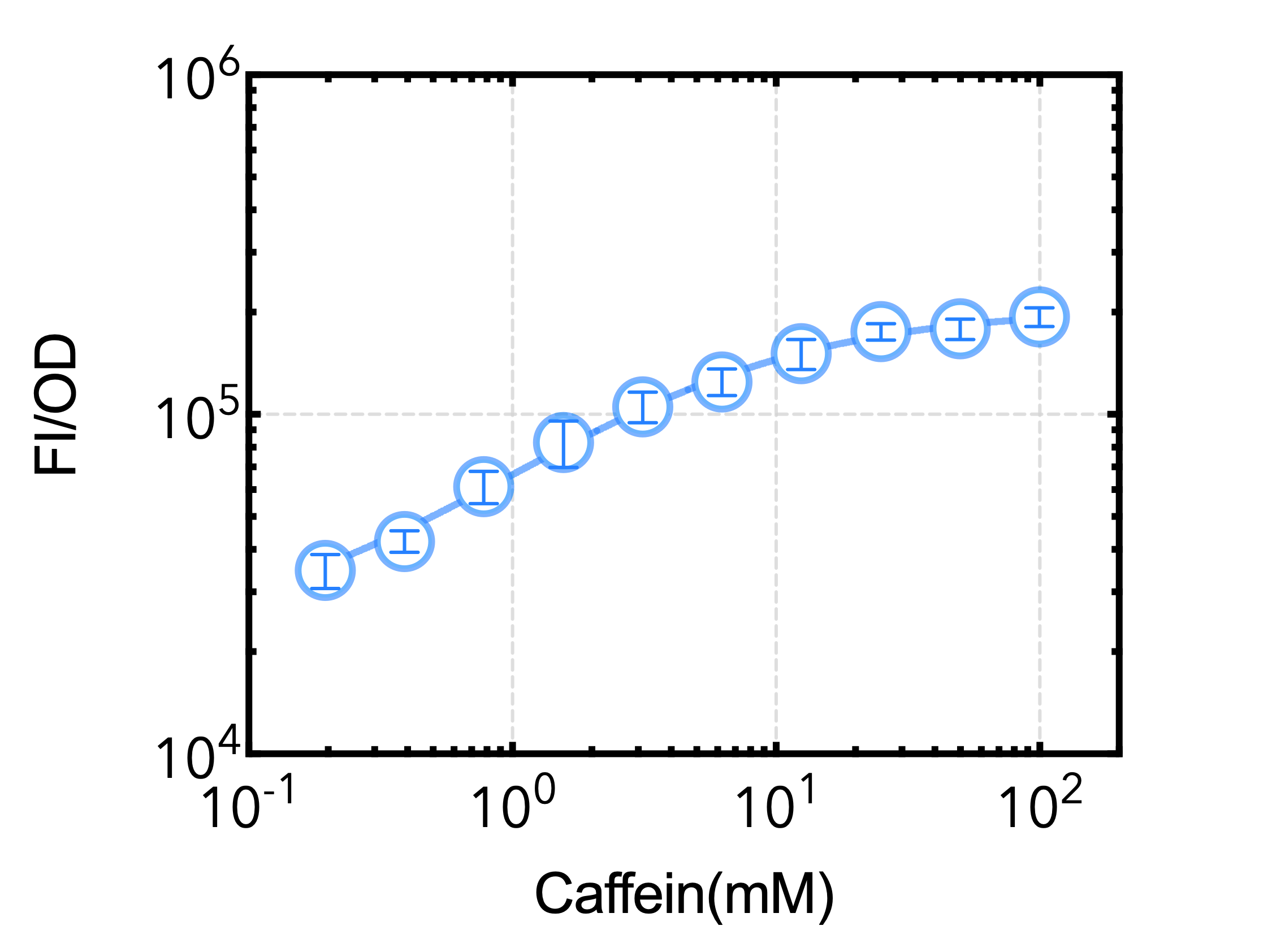Figure 2: The response curve of caffeine–controlled genetic switch. Samples prepared in triplicate, data represent the mean ± 1 s.d.

To analyze the performance of different designs, we fitted the sensors’ dose–response curves to a Hill function-based biochemical model to describe their input-output relationships.- The Hill constant EC50 is the inducer concentration that provokes half-maximal activation of a sensor; EC50 is negatively correlated with sensitivity.

- KTop is the sensor’s maximum output expression level; KTop is positively correlated with output amplitude.

From the data, we determined the following parameters：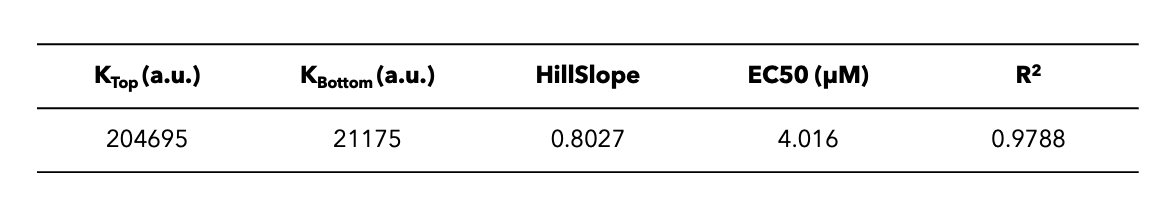## Matlab simulation### Results and analysis

As can be seen from the results of the Matlab analysis, the output of the caffeine sensor undergoes a rising at the beginning and then shows a decreasing trend after caffeine is completely consumed (Figure 4). Eventually, the output of the caffeine sensor gradually converges to 0 as the caffeine molecule is degraded or metabolized (Figure 3).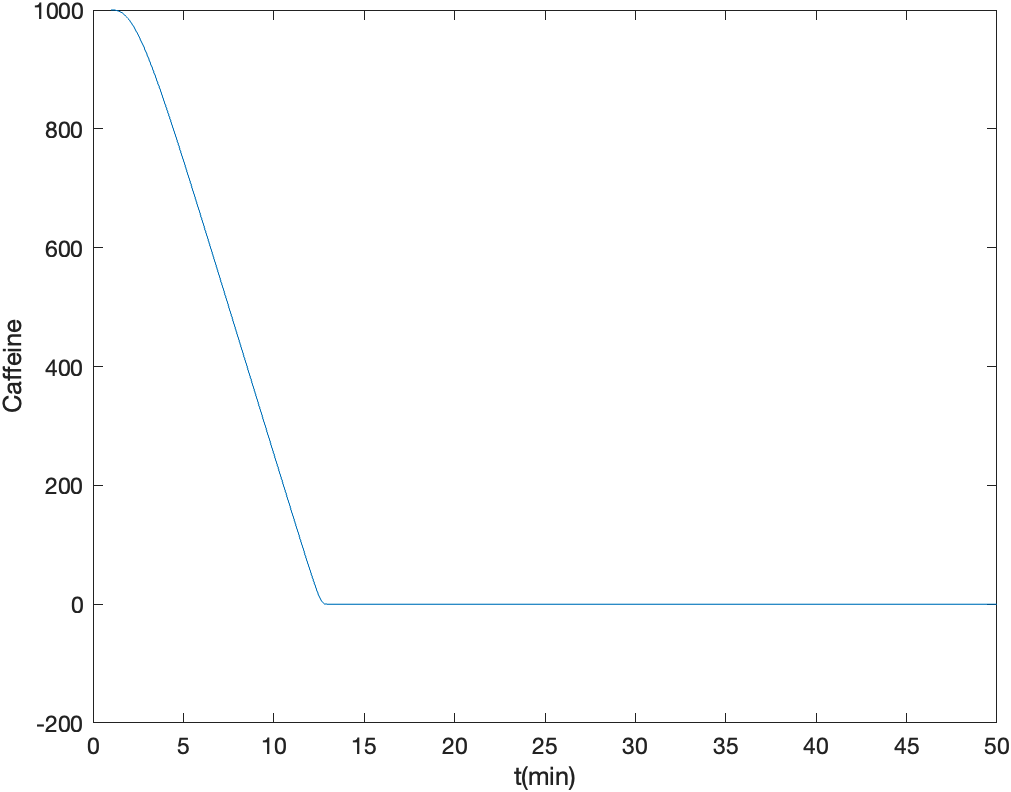Figure 3: Trends of consumption of Caffeine molecules over time.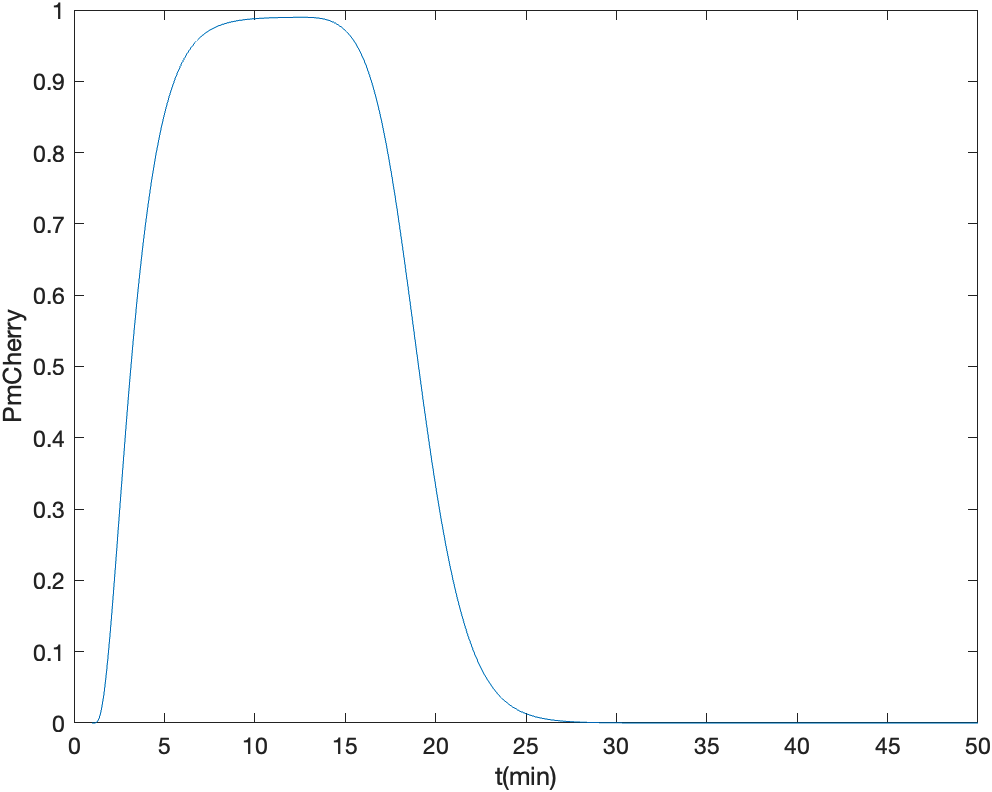Figure 4: Trend of relative fluorescence intensity of mCherry over time

In our project, we plan to use the Hidro system as a delivery device for the oral cavity or intestinal tract to achieve the expression of drug molecules or health regulatory molecules by referencing common beverages such as coffee. In these scenarios, the caffeine-sensing switch needs to be able to respond effectively to caffeine and eventually turn off the switch after caffeine metabolism is complete.

The results of our mathematical simulations meet this expectations, and the model developed here will have the potential to guide actual dietary inputs to achieve the desired health modulation effects.